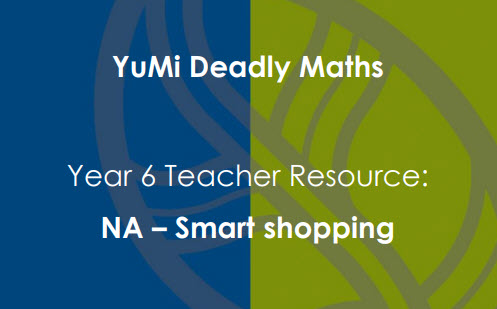# Smart shopping

Students compare and evaluate different shopping options.

Year level(s) Year 6
Audience Teacher
Purpose Teaching resource
Teaching strategies and pedagogical approaches Concrete Representational Abstract model, Culturally responsive pedagogies, Explicit teaching

## Curriculum alignment

Curriculum connections Numeracy
Strand and focus Number, Build understanding
Topics Fractions, Decimals, Percentage, Addition and subtraction, Money and financial mathematics
AC: Mathematics (V9.0) content descriptions
AC9M6N07
Solve problems that require finding a familiar fraction, decimal or percentage of a quantity, including percentage discounts, choosing efficient calculation strategies and using digital tools where appropriate

AC9M6N04
Apply knowledge of place value to add and subtract decimals, using digital tools where appropriate; use estimation and rounding to check the reasonableness of answers

AC9M6N09
Use mathematical modelling to solve practical problems, involving rational numbers and percentages, including in financial contexts; formulate the problems, choosing operations and efficient calculation strategies, and using digital tools where appropriate; interpret and communicate solutions in terms of the situation, justifying the choices made

Interpreting fractions (P7)
Multiplicative strategies (P8, P9)
Number and place value (P8)
Proportional thinking (P2)
Understanding money (P8)